What is the method for finding out what percent one fraction is of another fraction. For instance 4/5 is what percent of 1/9? I am in the 4th grade. Hi, Start by trying the same question with whole numbers rather than fractions. For example 20 is what percent of 50? I would do this in two steps First 20 is what fraction of 50? and then 20 is what percent of 50? The first step is 20 =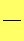50 whereis a fraction. You can make the equation above true by taking the fraction to be 20/50 = 2/5. Thus 20 is what 2/5 of 50? The second step is to change 2/5 to a percent. But a percent is just a fraction with 100 in the denominator so the second step is to complete the equation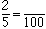5 times 20 is 100 thus multiply 2 times 20 to get 40. Hence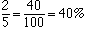Thus 20 is what 40% of 50? Now try fractions. 1/4 is what percent of 5/6? Step 1.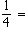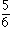This timeis 3/10. Step 2.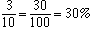Thus 1/4 is 30% of 5/6 Your problem was 4/5 is what percent of 1/9? (4/5 is larger than 1/9 so the answer will be more than 100%.) Try it. I got 4/5 is 720% of 1/9 Cheers, Penny Go to Math Central